Courses

# Test: Queuing Theory - 1

## 10 Questions MCQ Test Topicwise Question Bank for Mechanical Engineering | Test: Queuing Theory - 1

Description
This mock test of Test: Queuing Theory - 1 for Mechanical Engineering helps you for every Mechanical Engineering entrance exam. This contains 10 Multiple Choice Questions for Mechanical Engineering Test: Queuing Theory - 1 (mcq) to study with solutions a complete question bank. The solved questions answers in this Test: Queuing Theory - 1 quiz give you a good mix of easy questions and tough questions. Mechanical Engineering students definitely take this Test: Queuing Theory - 1 exercise for a better result in the exam. You can find other Test: Queuing Theory - 1 extra questions, long questions & short questions for Mechanical Engineering on EduRev as well by searching above.
QUESTION: 1

Solution:
QUESTION: 2

Solution:
QUESTION: 3

### In a queuing problem, if the arrivals are completely random, then the probability distribution of number of arrivals in a given time follows

Solution:

Explanation : Number of arrivals per unit time is estimated by the Poisson's distribution.

QUESTION: 4

If the number of arrival in a queue follows the poisson distribution, then the inter-arrival time obeys which one of the following distribution?

Solution:
QUESTION: 5

The arrival rate and the service time are usually assumed to respectively follow the

Solution:

Explanation : The arrival rate and the service time are usually assumed to respectively follow the binomial distribution and normal distribution.

QUESTION: 6

In a single server queuing model, the arrival rate is A, and the service rate is μ. What is the probability of system being idle?

Solution:
QUESTION: 7

In a single server queuing with arrival rate of λ and mean service time of μ, the expected number of customers in the system is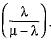What is the expected waiting time per customer in the system?

Solution:

Expected waiting time for the customer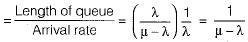QUESTION: 8

On an average, there are 30 customers in a queue. If the arrival rate of customers into the system is 16 customers per hour and on average 32 customers leave the system per hour, then the average number of customers in the system is

Solution: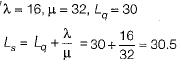QUESTION: 9

A repair shop is manned by a single worker. Customers arrive at the rate of 30 per hour. Time required to provide service is exponentially distributed with mean of 100 seconds. What is the mean waiting time of a customer, needing repair facility in the queue?

Solution:

λ = 30 per hour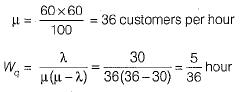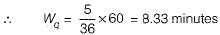QUESTION: 10

In the Kendall’s notation for representing queuing models the first position represents

Solution: